## Common Solids Questions and Answers - Form 1 Topical Mathematics

1. The figure below represents a square-based solid with a path marked on it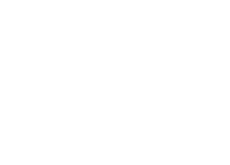Sketch and label the net of the solid
2. The below shows a solid prism:-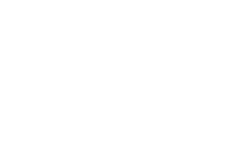1. Sketch the net of the prism above
2. Use the net in (a) above to calculate the total surface area of the material used in making the solid
3. Draw the solid whose net is shown below.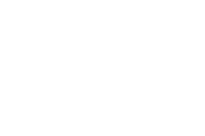4. Sketch the net of the solid shown in the figure below, measurements are in centimeters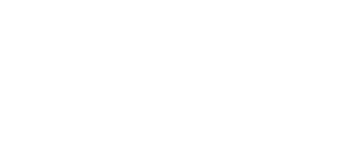1. Sketch of the net of the solid (not freehand) base n\must be square, other lengths must be within. Labeling of all verticals with the path correctly shown. AB and DA may be shown one.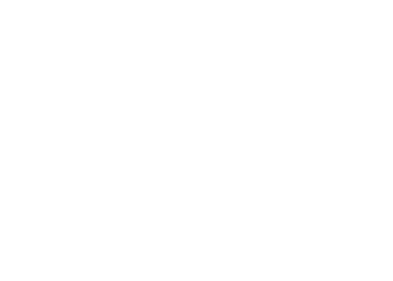1.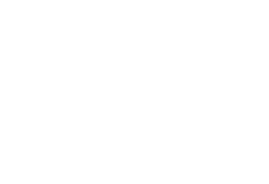2. Total surface area
= 2 √(9 x 3 x 4 x 2) + 10 (6 + 5 + 7)
= 29.39 + 180= 209.4cm
2.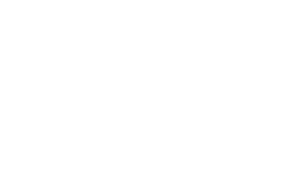3.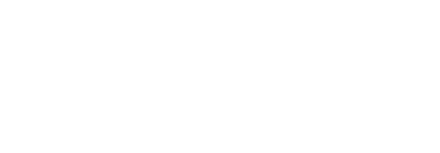• ✔ To read offline at any time.
• ✔ To Print at your convenience
• ✔ Share Easily with Friends / Students